# CREST Reasoning Olympiad Class 6 Sample Papers

## Syllabus:

Section 1: Series Completion and Inserting the Missing Character, Analogy and Classification, Blood Relations, Coding - Decoding, Direction Sense Test, Logical Venn Diagram, Alpha Numeric Sequence Puzzle, Number, Ranking and Time Sequence Test, Logical Sequence of Words, Alphabet Test, Mathematical Operations, Analytical Reasoning, Mirror Images and Water Images, Embedded Figures, Figure Formation, Construction of Squares, Grouping of Identical Figures, Figure Matrix, Paper Folding and Paper Cutting, Cubes and Dice, Dot Situations.

Achievers Section: Higher Order Thinking Questions - Syllabus as per Section 1

 Q.1 Two positions of a dice are shown below: When 2 is at the bottom, what number will be at the top?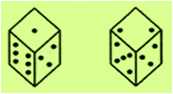Q.2 A solid cube of each side 8 cm, has been painted red, blue and black on pairs of opposite faces. It is then cut into cubical blocks of each side 2 cm. (Answer the following: How many cubes have two faces painted black?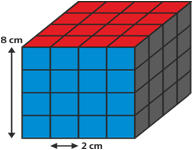Q.3 In each of the following questions : find out which of the answer figures (1), (2), (3) and (4) completes the figure matrix? Select a suitable figure from the four alternatives that would complete the figure matrix.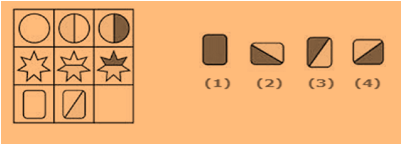Q.4 If Mumbai is called Delhi, Delhi is called Bangalore, Bangalore is called Gujarat, then where is the Red Fort located?
 Q.5 In a dice a, b, c and d are written on the adjacent faces, in a clockwise order and e and f at the top and bottom.If c is at the top, which alphabet is at the bottom ?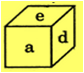Q.6 A dice is numbered from 1 to 6 in different ways. If 1 is opposite to 2 and adjacent to 3 and 4, then which of the following statements is necessarily true?
 Q.7 In each of the following questions : find out which of the answer figures (1), (2), (3) and (4) completes the figure matrix? Select a suitable figure from the four alternatives that would complete the figure matrix.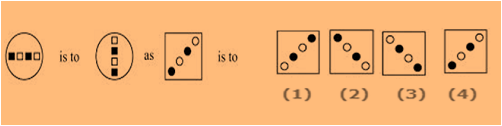Q.8 Each of the following questions consists of a set of three figures X, Y and Z shows a sequence of folding of apiece of paper. Figure (Z) shows the manner in which the folded paper has been cut. These three figures are followed by four answer figures from which you have to choose a figure which would most closely resemble the unfolded form of figure (Z).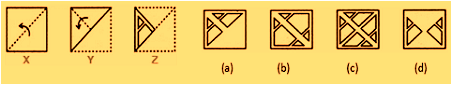Q.9 If black means orange, orange means red, red means brown, then what is the colour of Apple?
 Q.10 Select a suitable figure from the four alternatives that would complete the figure matrix:-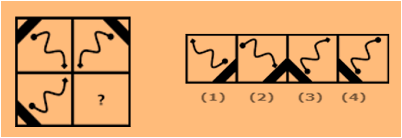Sample PDF of CREST Reasoning Olympiad for Class 6: